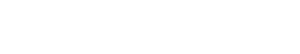# Median

The median is a measure of central tendency that is used to split a data set into two equal halves. It is often used as a way to summarize data and to find the average value in a data set. The median can be calculated for both quantitative and categorical data.

For quantitative data, the median is the middle value when the values are sorted in order from least to greatest. If there is an even number of values, then the median is the mean of the two middle values. For categorical data, the median is the category that has an equal number of observations above and below it.

The median is sometimes used as a measure of central tendency because it is not affected by outliers or extreme values. This can be a disadvantage as well because it means that the median is not a very good measure of spread.

The median is also known as the 50th percentile because it splits the data set in half. The 25th percentile is known as the first quartile, and the 75th percentile is known as the third quartile. These percentiles are used to split the data set into four equal parts.

## How to Solve Median

There are a few different ways to solve median problems. The most common way is to use the median function on a calculator. This will give you the exact answer.

If you don’t have a calculator, you can still solve median problems by finding the middle value of the data set. To do this, you need to put the values in order from least to greatest and then find the value in the middle. If there are an even number of values, then you take the mean of the two middle values.

You can also use estimation to solve median problems. This is often faster than using a calculator or finding the middle value, but it is not as accurate. To estimate the median, you find a value that is close to the middle of the data set.Unlock the power of actionable insights with AI-based natural language processing.